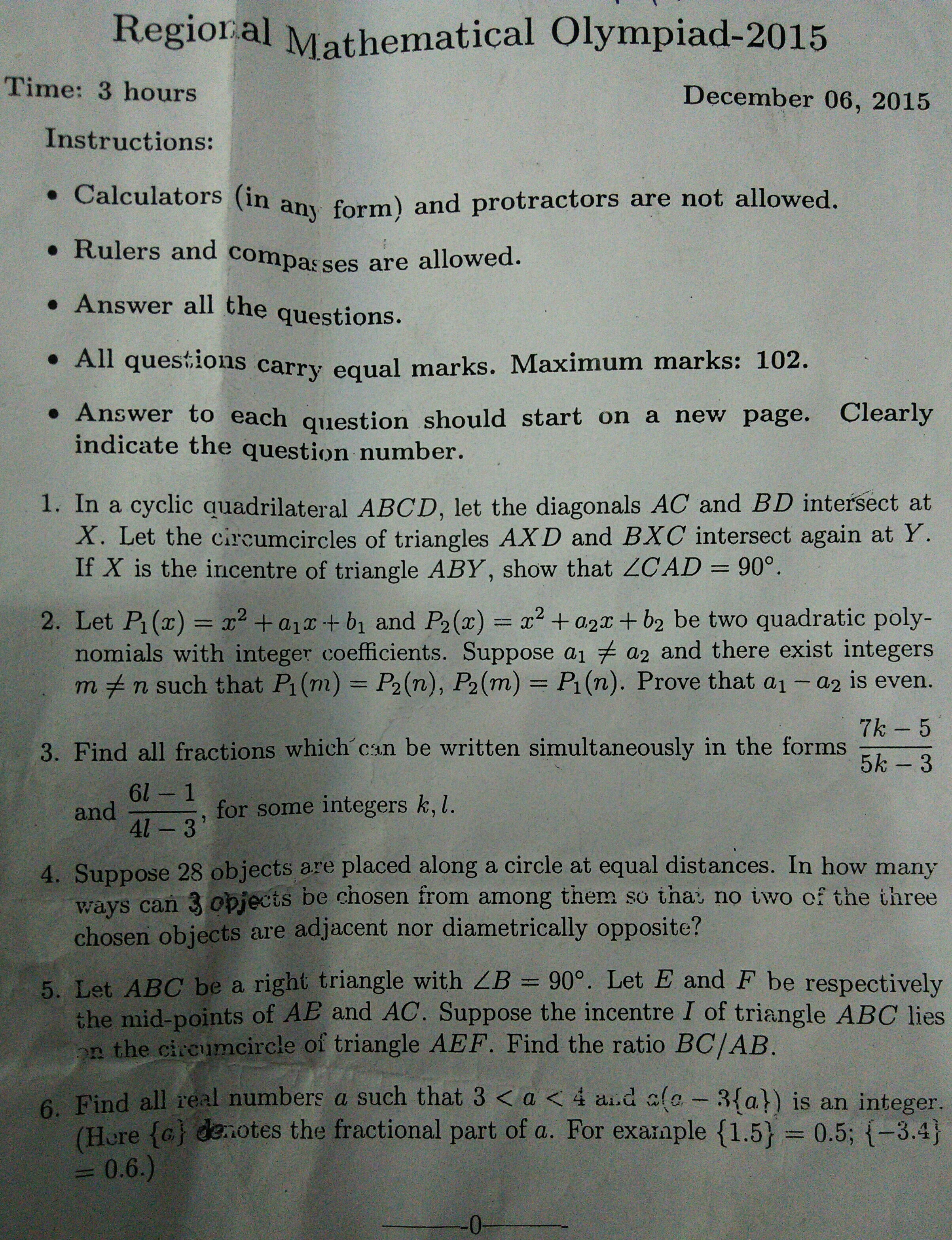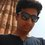# CBSE GMO 2015 Paper discussionGroup Mathematical Olympiad is conducted by CBSE . It is only for CBSE students . Every CBSE school can send 5 students for it. Around 30 students are selected from the country and are eligible to write INMO.Note by Aditya Chauhan
5 years, 6 months ago

This discussion board is a place to discuss our Daily Challenges and the math and science related to those challenges. Explanations are more than just a solution — they should explain the steps and thinking strategies that you used to obtain the solution. Comments should further the discussion of math and science.

When posting on Brilliant:

• Use the emojis to react to an explanation, whether you're congratulating a job well done , or just really confused .
• Ask specific questions about the challenge or the steps in somebody's explanation. Well-posed questions can add a lot to the discussion, but posting "I don't understand!" doesn't help anyone.
• Try to contribute something new to the discussion, whether it is an extension, generalization or other idea related to the challenge.
• Stay on topic — we're all here to learn more about math and science, not to hear about your favorite get-rich-quick scheme or current world events.

MarkdownAppears as
*italics* or _italics_ italics
**bold** or __bold__ bold
- bulleted- list
• bulleted
• list
1. numbered2. list
1. numbered
2. list
Note: you must add a full line of space before and after lists for them to show up correctly
paragraph 1paragraph 2

paragraph 1

paragraph 2

[example link](https://brilliant.org)example link
> This is a quote
This is a quote
    # I indented these lines
# 4 spaces, and now they show
# up as a code block.

print "hello world"
# I indented these lines
# 4 spaces, and now they show
# up as a code block.

print "hello world"
MathAppears as
Remember to wrap math in $$ ... $$ or $ ... $ to ensure proper formatting.
2 \times 3 $2 \times 3$
2^{34} $2^{34}$
a_{i-1} $a_{i-1}$
\frac{2}{3} $\frac{2}{3}$
\sqrt{2} $\sqrt{2}$
\sum_{i=1}^3 $\sum_{i=1}^3$
\sin \theta $\sin \theta$
\boxed{123} $\boxed{123}$

## Comments

Sort by:

Top Newest

Answer to question 3. $\frac{6l-1}{4l-3}=\frac{7k-5}{5k-3}$ After cross multiplication we get $8k+l+lk=6$ Adding 8 to both sides $8k+8+l+lk=14$ $8(k+1)+l(1+k)=14$ $(8+l)(1+k)=14$ Since $l,k$ are integers therefore the solutions of $(l,k)=(-1,1),(-6,6),(6,0),(-7,13),(-15,-3),(-22,-2),(-10,-8),(-9,-15)$ Therefore the required fractions are $1,\frac{43}{31},\frac{5}{3},\frac{37}{27},\frac{13}{9},\frac{19}{13},\frac{61}{43},\frac{55}{39}$

- 5 years, 6 months ago

Log in to reply

Can anybody please post a proper solution for question $4$ with explanation?

- 5 years, 5 months ago

Log in to reply

Number of ways of selecting points from points is 28C3=3276

Now, we will eliminate some conditions..

Number of ways of selecting all 3 as adjacent points is 28.

Number of ways of selecting 2 adjacent points and one not adjacent with them is 28×24=672

Number of ways of selecting two points opposite diametrically along with the third point not adjacent to the former points is 14×22=308.

Hence, the desired result will be 3276-28-672-308=2268

- 5 years, 5 months ago

Log in to reply

1 will be rejected as it isn't a fraction.

- 3 years, 8 months ago

Log in to reply

Answer to question number 2. $P_{1}(m)-P_{2}(n)=a_{1}-a_{2}=\frac{2(b_{2}-b_{1})}{m-n}$ This implies that $a_{1}-a_{2}=even$. $P_{1}(m)-P_{2}(n)=a_{1}+a_{2}=-2(m+n)$ This implies that $a_{1}+a_{2}=even$. Hence proved.

- 5 years, 6 months ago

Log in to reply

Shivam dud you gave GMO or RMO?

- 5 years, 6 months ago

Log in to reply

RMO

- 5 years, 6 months ago

Log in to reply

How many did you solve?

- 5 years, 6 months ago

Log in to reply

5

- 5 years, 6 months ago

Log in to reply

when will the results of gmo will be declared

- 5 years, 5 months ago

Log in to reply

Can anyone tell the answer of 5th question

- 5 years, 6 months ago

Log in to reply

it is 4/3

- 5 years, 5 months ago

Log in to reply

I m getting 1/3

- 5 years, 5 months ago

Log in to reply

see i can't post my solution because it is too long but you can verify it by construction.

- 5 years, 5 months ago

Log in to reply

I was able to do 4.5 questions do i stand a chance to get selected...

- 5 years, 6 months ago

Log in to reply

Is the answer to question 4 2268?

- 5 years, 6 months ago

Log in to reply

Yes it is

- 5 years, 6 months ago

Log in to reply

Can you tell me what is the expected cutoff for gmo?

- 5 years, 6 months ago

Log in to reply

Answer to question 6. Let $a=m+\frac{b}{c}$ where $m$ is any integer and $0 . Then $a(a-3{a})=( m+\frac{b}{c})( m-2\frac{b}{c})$ $m^{2}-\frac{bm}{c}+\frac{2b^{2}}{c^{2}}$. $m^{2}-\frac{2b^{2}-bcm}{c^{2}}$ Now, $m$ is an integer . Let's consider $\frac{2b^{2}-bcm}{c^{2}}=k$ where $k$ is an integer . After solving we get $\frac{b}{c}=\frac{m+_{-}\sqrt{m^{2}+8k}}{4}$.....(I) But $\frac{b}{c}<1$....(II) Now putting value of $\frac{b}{c}$ from (I) to (II). We get $m+k<2$ Therefore there are infinitely many integers $m,k$ such that $m+k<2$. Hence proved.

- 5 years, 6 months ago

Log in to reply

Bro ur equation which is quadratic in m is wrong also we will get 4 values of a

- 5 years, 6 months ago

Log in to reply

A general solution $a=(2n+1)+0.5$ where $n$ is a integer.

- 5 years, 6 months ago

Log in to reply

@Shivam Jadhav This ques is a little different from the RMO one , we have to find all values of 'a' between 3 & 4. @Devansh Shah is right , there will be 4 values.

- 5 years, 6 months ago

Log in to reply

m cant be 'any' integer as 3<a<4. So a will be 3.something or a = 3 + b/c

- 5 years, 6 months ago

Log in to reply

you can try the 6 question posted by me they all are of gmo.

- 5 years, 5 months ago

Log in to reply

×

Problem Loading...

Note Loading...

Set Loading...